### Bisected Angle

Illustration to show that if an angle is bisected, and if a line drawn through the vertex perpendicular…### Bisected Angles

Illustration to show that the bisectors of two supplementary adjacent angles are perpendicular to each…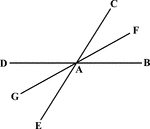### Bisected Angle

Illustration to show that the bisector of one of two vertical angles bisects the other.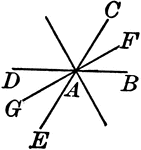### Bisected Angles

Illustration to show that the bisector of two pairs of vertical angles formed by two intersecting lines…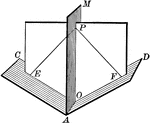### Dihedral Angle With Bisector

Illustration of a dihedral angle bisected by a plane. "Every point in a plane which bisects a dihedral…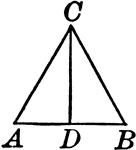### Bisected Vertical Angle of an Isosceles Triangle

Illustration to show that the bisector of the vertical angle of an isosceles triangle bisects the base,…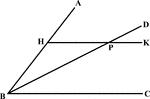### Angle Bisector Drawn Parallel to A Side

Illustration to show that if through any point in the bisector of an angle a line is drawn parallel…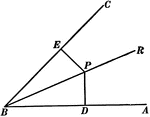### Perpendiculars To The Sides Of An Angle

Illustration used to show "The two perpendiculars to the sides of an angle from any point in its bisector…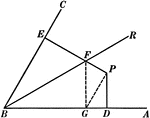### Perpendiculars To The Sides Of An Angle

Illustration used to show "The two perpendiculars to the sides of an angle from any point not in its…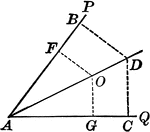### Angle Bisector

Illustration showing that the bisector of a given angle is the locus of points equidistant from the…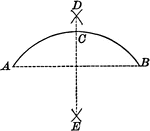### Construction of Bisecting a Given Arc

Illustration of the construction used to bisect a given arc.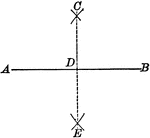### Construction of Bisecting a Given Line

Illustration of the construction used to bisect a given line.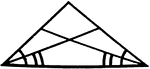### Angle Bisectors In An Isosceles Triangle

Illustration showing that if two angles of a triangle are equal, the bisectors of these angles are equal.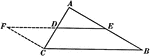### Line Joining Midpoints on a Triangle

Illustration used to prove "The line joining the mid-points of two sides of a triangle is parallel to…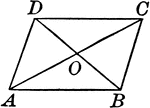Illustration to show that if the diagonals of a quadrilateral bisect each other, the figure is a parallelogram.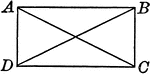### Rectangle With Diagonals

Illustration to show that if the diagonals of a parallelogram are equal, the figure is a rectangle.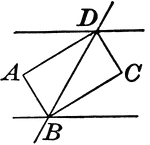### Rectangle Formed When Two Parallel Lines are Cut by a Transversal

Illustrations to show that if two parallel lines are cut by a transversal, the bisectors of the interior…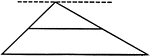### Line Bisecting Side of a Triangle

Illustration used to prove "The line bisecting one side of a triangle and parallel to another side bisects…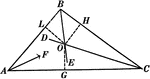### Angle Bisectors In A Triangle

Illustration used to prove "The bisectors of the angles of a triangle are concurrent in a point which…### Bisector of an Exterior Angle of an Isosceles Triangle

Illustration to show that the bisector of an exterior angle of an isosceles triangle, formed by producing…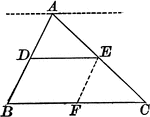### Triangle With Bisector Parallel to the Base

Illustration of a triangle showing that when a line parallel to the base of a triangle bisects one side,…### Circumcenter of Triangle

Illustration to show perpendicular bisectors in a triangle. It is known as the circumcenter.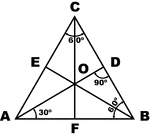### Equilateral Triangle

Sketch of equilateral triangle with bisectors, medians, and altitudes.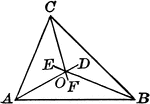### Incenter of Triangle

Illustration to show angle bisectors in a triangle. It is known as the incenter.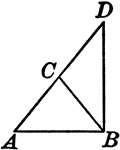### Leg Produced through the Vertex of an Isosceles Triangle

Illustration to show that if one of the legs of an isosceles triangle is produced through the vertex…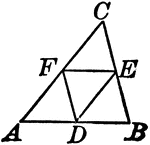### Midpoints of Triangle Divide Triangle into Four Equal Triangles

Illustration to show that if the lines joining the middle points of the sides of a triangle divide the…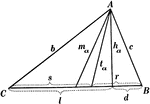### Parts Of A Triangle

Illustration used to show the various parts of a triangle: sides, angles, medians, altitudes, bisectors,…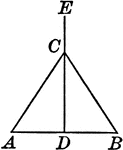### Perpendicular Bisector of the Base of an Isosceles Triangle

Illustration to show that the perpendicular bisector of the base of an isosceles triangle passes through…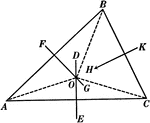### Perpendicular Bisectors In A Triangle

Illustration used to prove "The perpendicular bisectors of the sides of a triangle are concurrent in…